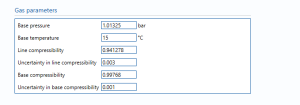# Gas PTZ

## Gas ParametersBase pressure – The pressure at standard conditions.

Base temperature – The temperature at standard conditions.

Line compressibility – the compressibility of the flowing gas.

Note: compressibility is the correction factor for the deviation of the real gas from ideal gas.

uncertainty in line compressibility – the uncertainty in the compressibility value.

Base compressibility – the compressibility of gas at standard conditions.

Note: compressibility is the correction factor for the deviation of the real gas from ideal gas.

uncertainty in base compressibility – the uncertainty in the compressibility value at standard conditions.

### Uncertainty Budget

The uncertainty budget table shows a break down of the different components that contribute to the overall calculated uncertainty.The values input into the uncertainty budget are derived from the measured pressure and temperature transmitter specific values relating to its calibration and specification. These values are taken in as the expanded uncertainties and are divided by a coverage factor to gain the standard uncertainty.

The coverage factor is determined by the probability distribution that best suits that uncertainty component. The standard uncertainty is then multiplied by the sensitivity value then squared. This is done for each component that contributes to the overall uncertainty in Volume Correction Factor (VCF). The Standard Uncertainty in VCF is the square root of the sum of each component variance as shown in the following equation:### Calculated UncertaintyThe Expanded Uncertainty is the Standard Uncertainty multiplied by the coverage factor (k). The coverage factor is defaulted to k = 2 (equivalent to a confidence level of approximately 95%).

The Relative Uncertainty is the Expanded Uncertainty divided by VCF.

Back to Uncertainty Modules